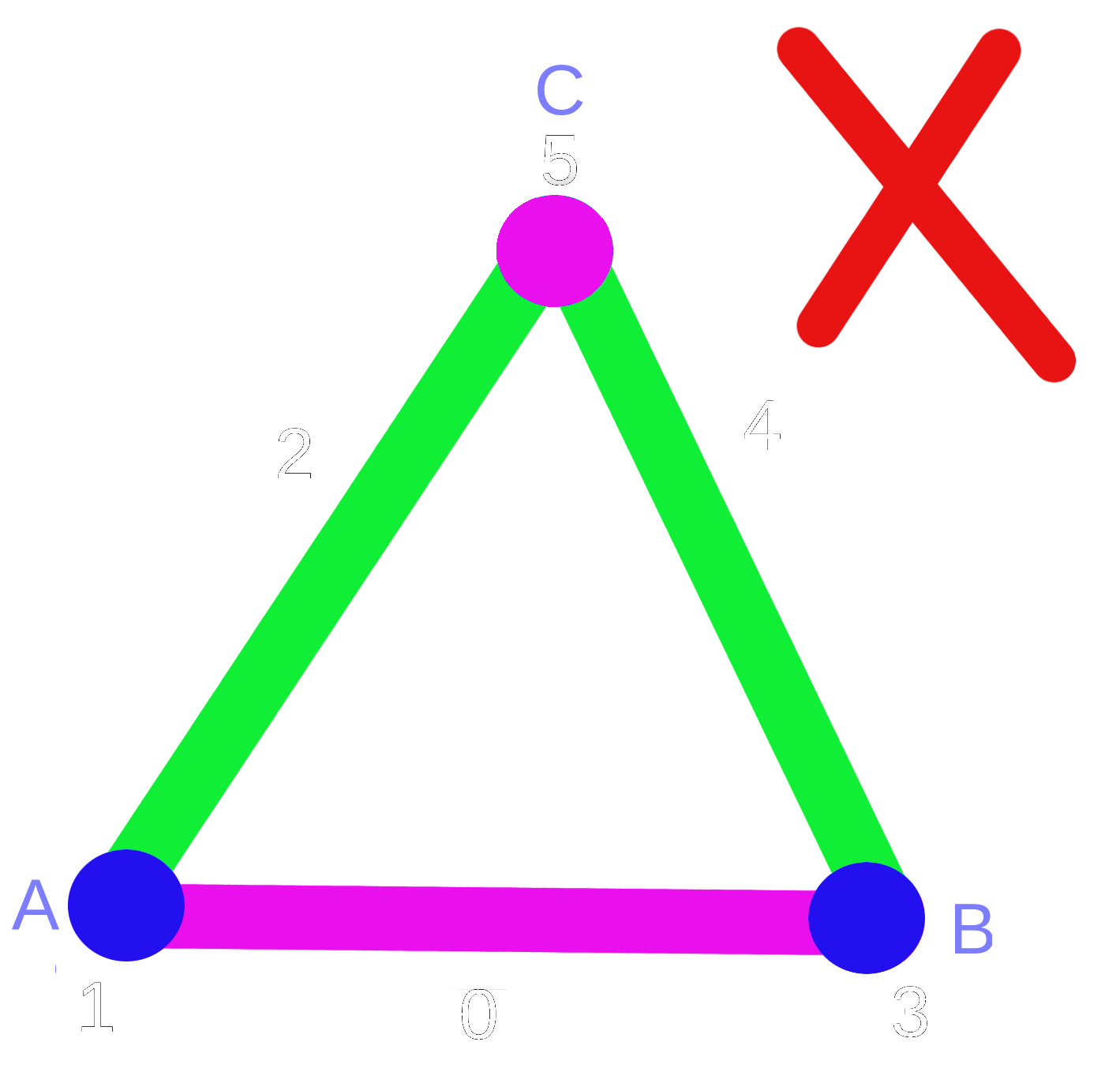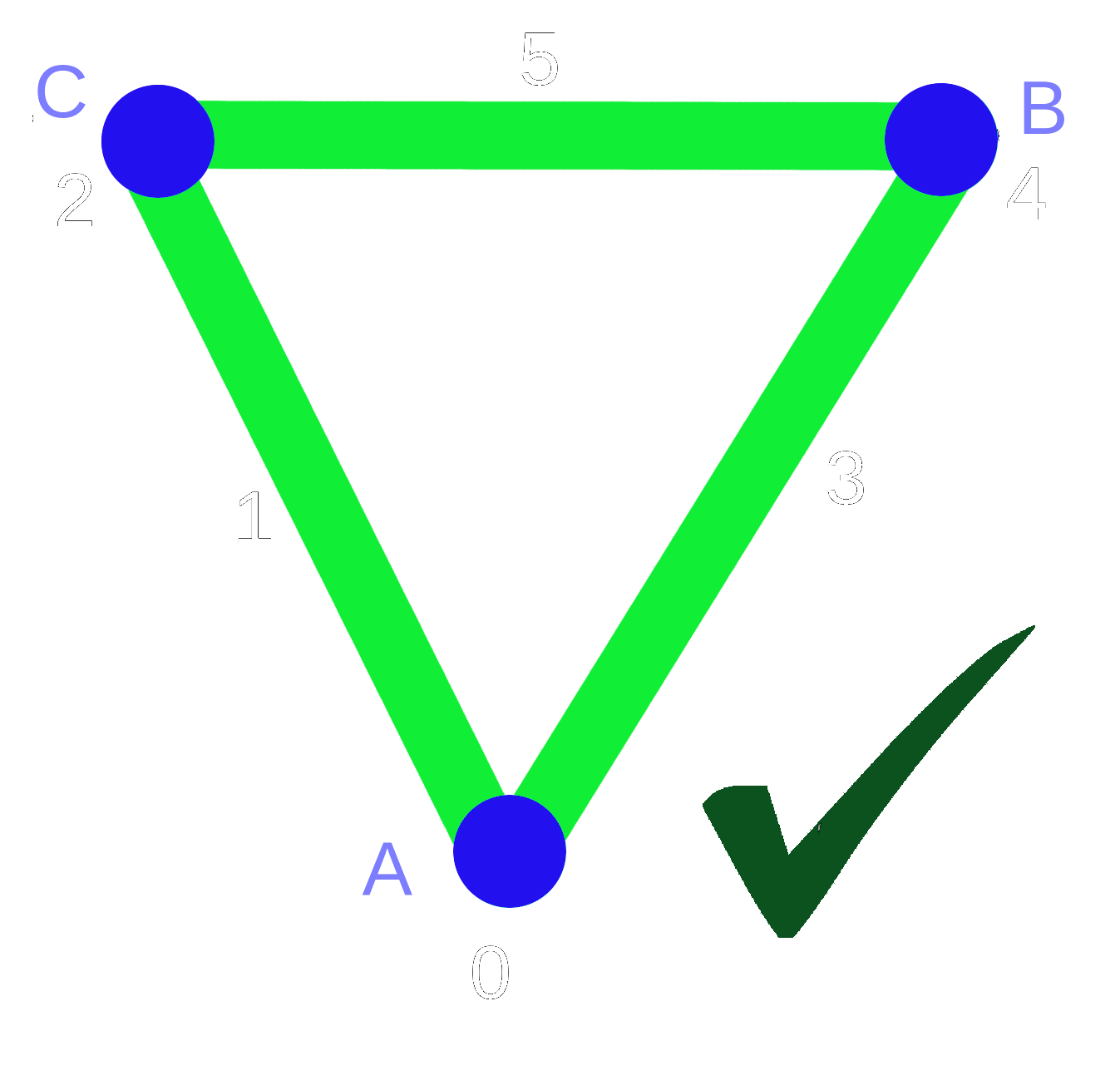# Simplicial Complex

To represent topological spaces in computer, we need to discretize them. For this purpose, we use the simplicies. A simplex is a generalization of the notion of a triangle or tetrahedron to arbitrary dimensions. For example, a 0-simplex is a point, a 1-simplex is a line segment, a 2-simplex is a triangle, a 3-simplex is a tetrahedron

Every simplex with dimension > 0 has faces. A simplex β with dimension d has d+1 faces with dimension d-1. To understand this, we use the following concrete examples.e, which is 2-dimensional simplex (edge), has two faces: V1 and V2 . e is cofaces of V1 and V2t, which is 3-dimensional simplex, has three faces: e1 , e2 and e3 and t is called coface for e1, e2 and e3 The notion of the face is "<", so in the first example,we write V1 < e and we say V1 is a face of e. The notion of coface is ">" and we write e > V1 and this means e is coface of V1.
Now, we want to give the main definiton of this section. A simplicial complex $\mathcal{K}$ is a set of simplices that satisfies the following conditions:
1. Every face of a simplex from $\mathcal{K}$ is also in $\mathcal{K}$.
2.The non-empty intersection of any two simplices $\sigma_1,\sigma_2 \in \mathcal{K}$ is a face of both $\sigma_1$ and $\sigma_2$.

# Triangulation

A triangulation of topological space X is a simplicial complex $\mathcal{K}$ with a homeomorphism $h: \mathcal{K}\rightarrow X$. If we take the torus as an example of a topological space, we can find many triangulation of it, but in the next image we see the triangulation of the topological spaces with small number of simplices.Triangulation of torus Note that the simplices labeled with the same numbers are the same simplices.

# Discrete Morse Function

Let $\mathcal{K}$ be a simplicial complex. A function $f:\mathcal{K}\rightarrow \mathbb{R}$ is a discrete Morse function if:
1. The number of $\{\beta^{(p+1)}>\alpha^p \mid f(\beta) \leq f(\alpha)\}\leq 1$
2. The number of $\{\gamma^{(p-1)}<\alpha^p \mid f(\gamma) \geq f(\alpha)\}\leq 1$
To understand the definition, we look at a counter exampleNot a Morse Function In the above image, we have a 1-d simplicial complex, the function defined on the simplices of the complex is not discrete Morse function. Why? because the 0-simplex (point) C has function value = 5 but it hase 2 cofaces with less function value, namely the edge CA and the edge CB. This contradicts the first condition of the definiton. Also, the edge AB has function value =0 and this is greater than 2 of its faces, namely the point A and point B, this contradicts the second condition of the definiton.
In the next example, the 1-d simplicial complex has discrete Morse function because the two conditions of the definiton are fulfilled.Morse Function The other main ingredient in discrete Morse Theory is the notion of a critical point.
Let $\mathcal{K}$ be a simplicial complex and f is a discrete Morse function, we say that a simplex in $\mathcal{K}$ is critical if:
1.The number of $\{\beta^{(p+1)}>\alpha^p \mid f(\beta) \leq f(\alpha)\}=0$
2.The number of $\{\beta^{(p+1)}>\alpha^p \mid f(\beta) \leq f(\alpha)\}=0$
If we look at the discrete Morse function in the last figure we can recognize two critical cells . The first critical cell is the point A because A has no coface with smaller or equal function value and has no faces with greater function value. The second critical cell is the edge BC because all the faces of BC have less function value and BC has no cofaces.

If we have a simplicial complex with discrete Morse function f, we know from the definiton that every simplex $\beta$ has at maximum one face $\alpha$ with $f(\alpha) \geq f(\beta)$. If we draw an arrow from $\alpha$ to $\beta$ (the tail on $\alpha$ and the head on $\beta$) this will lead us to the fact that every simplex $\alpha$ in the complex is one of the following:
1. $\alpha$ is the tail of exactly one arrow.
2. $\alpha$ is the head of exactly one arrow.
3. $\alpha$ is neither the tail nor the head of any arrow.Drawing Arrows Now if we have a simplicial complex and a drawing in arrows like we exaplained previously, one can suppose that this drawing corresponds to a discrete Morse function. The drawn arrow from $\alpha$ to $\beta$ can be mathematically written as $\{ \alpha^p <\beta^{p+1}\}$ and we call it pair. The collection of pairs in a simplicial complex is called gradient vector field. A gradient vector field V on a simplicial complex $\mathcal{K}$ is a collection of pairs $\{ \alpha^p <h\beta^{p+1}\}$ of simplices of $\mathcal{K}$ such that every simplex is in at most one pair of V.